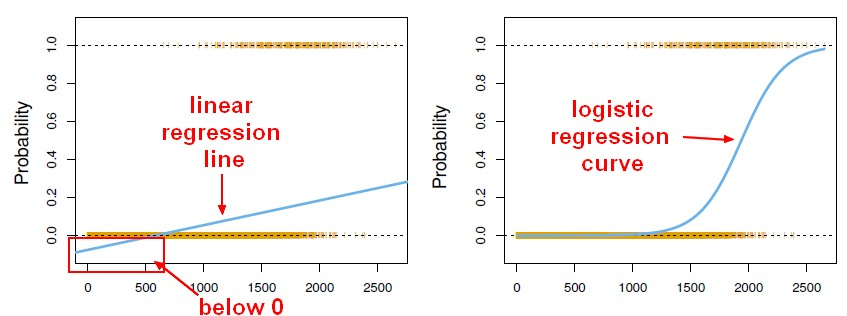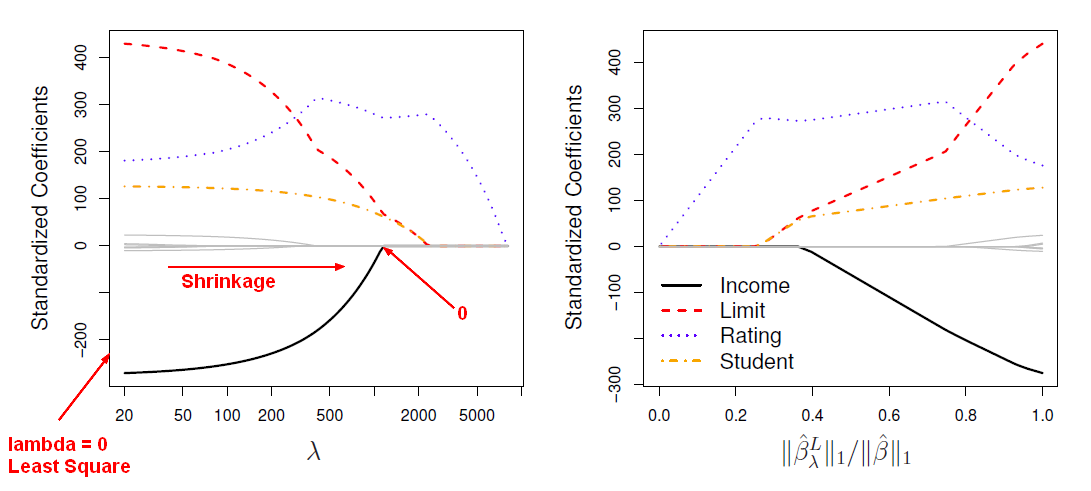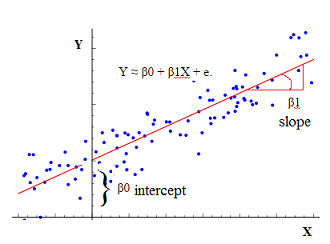# Statistics - Residual sum of Squares (RSS) = Squared loss ?

The Residual sum of Squares (RSS) is defined as below and is used in the Least Square Method in order to estimate the regression coefficient.

The smallest residual sum of squares is equivalent to the largest r squared.

The deviance calculation is a generalization of residual sum of squares.

Squared loss = $(y-\hat{y})^2$

## Equation

$$\begin{array}{rrl} \text{Residual sum of Squares (RSS)} & = & \sum_{i=1}^{\href{sample_size}{N}}(\href{residual}{residual})^2 \\ RSS & = & \sum_{i=1}^{\href{sample_size}{N}}(\href{residual}{e_i})^2 \\ RSS & = & \sum_{i=1}^{\href{sample_size}{N}}(Y_i-\hat{Y_i})^2 \\ \end{array}$$

where:

The residual sum is squared to get rid of the negative sign.

## Example

### Simple Regression

$$\begin{array}{rrl} RSS & = & \sum_{i=1}^{\href{sample_size}{N}}(Y_i-\hat{B}_0-\hat{B}_1 X_i)^2 \\ \end{array}$$

### Multiple Regression

$$\begin{array}{rrl} RSS & = & \sum_{i=1}^{\href{sample_size}{N}}(Y_i-\hat{B}_0-\hat{B}_1 X_{i1}-\dots-\hat{B}_n X_{in})^2 \\ & = & \sum_{i=1}^{\href{sample_size}{N}}(Y_i-\hat{B}_0-\sum_{j=1}^{\href{dimension}{P}}\hat{B}_j X_{ij})^2 \\ \end{array}$$

Recommended PagesMachine Learning - (Univariate|Simple) Logistic regression

A Simple Logistic regression is a Logistic regression with only one parameters. For the generalization (ie with more than one parameter), see Logistic regression comes from the fact that linear regression...R - Feature Selection - Indirect Model Selection

In a feature selection process, once you have generated all possible models, you have to select the best one. This article talks the indirect methods. We will select the models using CP but as...R - Feature selection - Model Generation (Best Subset and Stepwise)

This article talks the first step of feature selection in R that is the models generation. Once the models are generated, you can select the best model with one of this approach: Best...Statistical Learning - Lasso

Lasso is a shrinkage method. Ridge regression doesn't actually select variables by settings the parameters to zero. Lasso is a more recent technique for shrinking coefficients in regression that overcomes...Statistics - (No Predictor|Mean|Null) Model

The simplest prediction in a regression that you can imagine is: using the mean of the target () as prediction ie choosing a slope of 0 The mean model is also known as the “No Model”. With...Statistics - (Univariate|Simple|Basic) Linear Regression

A Simple Linear regression is a linear regression with only one predictor variable (X). Correlation demonstrates the relationship between two variables whereas a simple regression provides an equation...A big R squared indicates a model that really fits the data well. But unfortunately, you can't compare models of different sizes by just taking the one with the biggest R squared because you can't compare...Statistics - Akaike information criterion (AIC)

AIC stands for Akaike Information Criterion. Akaike is the name of the guy who came up with this idea. AIC is a quantity that we can calculate for many different model types, not just linear models,...Statistics - Best Subset Selection Regression

The most direct approach in order to generate a set of model for the feature selection approach is called all subsets or best subsets regression. We compute the least squares t for all possible subsets...Statistics - Deviance

The deviance is negative two times the maximized log-likelihood. And in the case of least squares regression, the deviance and the residual sum of squares are equivalent but for other model types the...